Effects of Material Characteristics on Nonlinear Dynamics of Viscoelastic Axially Functionally Graded Material Pipe Conveying Pulsating Fluid

Guangming Fu, Yuhang Tuo, Heen Zhang, Jian Su, Baojiang Sun, Kai Wang, Min Lou (2023). Effects of Material Characteristics on Nonlinear Dynamics of Viscoelastic Axially Functionally Graded Material Pipe Conveying Pulsating Fluid. Journal of Marine Science and Application, 22(2): 247-259. https://doi.org/10.1007/s11804-023-00328-8
 Citation: Guangming Fu, Yuhang Tuo, Heen Zhang, Jian Su, Baojiang Sun, Kai Wang, Min Lou (2023). Effects of Material Characteristics on Nonlinear Dynamics of Viscoelastic Axially Functionally Graded Material Pipe Conveying Pulsating Fluid. Journal of Marine Science and Application, 22(2): 247-259. https://doi.org/10.1007/s11804-023-00328-8## Effects of Material Characteristics on Nonlinear Dynamics of Viscoelastic Axially Functionally Graded Material Pipe Conveying Pulsating Fluid

##### https://doi.org/10.1007/s11804-023-00328-8
Funds:

the National Natural Science Foundation of China 52171288

the National Natural Science Foundation of China 51890914

the Key Research and Development Program of Shandong Province (Major Innovation Project) 2022CXGC020405

the National Ministry of Industry and Information Technology Innovation Special Project—Engineering Demonstration Application of Subsea Oil and Gas Production SystemSubject 4 “Research on Subsea Christmas Tree and Wellhead Offshore Testing Technology” MC-201901-S01-04

the Fundamental Research Funds for the Central Universities 20CX02410A

the Development Fund of Shandong Key Laboratory of Oil & Gas Storage and Transportation Safety, and CNPq, CAPES and FAPERJ of Brazil

###### Corresponding author: Guangming Fu, fu@upc.edu.cn
• Abstract

The nonlinear dynamic behaviors of viscoelastic axially functionally graded material (AFG) pipes conveying pulsating internal flow are very complex. And the dynamic behavior will induce the failure of the pipes, and research of vibration and stability of pipes becomes a major concern. Considering that the elastic modulus, density, and coefficient of viscoelastic damping of the pipe material vary along the axial direction, the transverse vibration equation of the viscoelastic AFG pipe conveying pulsating fluid is established based on the Euler-Bernoulli beam theory. The generalized integral transform technique (GITT) is used to transform the governing fourth-order partial differential equation into a nonlinear system of fourth-order ordinary differential equations in time. The time domain diagram, phase portraits, Poincaré map and power spectra diagram at different dimensionless pulsation frequencies, are discussed in detail, showing the characteristics of chaotic, periodic, and quasi-periodic motion. The results show that the distributions of the elastic modulus, density, and coefficient of viscoelastic damping have significant effects on the nonlinear dynamic behavior of the viscoelastic AFG pipes. With the increase of the material property coefficient k, the transition between chaotic, periodic, and quasi-periodic motion occurs, especially in the high-frequency region of the flow pulsation.

Article Highlights
• The transverse vibration equation is established for the viscoelastic axially functionally graded material (AFG) pipe conveying pulsating fluid.
• The generalized integral transform technique is developed to analyze the nonlinear dynamics of AFG pipes.
• The effects of the elastic modulus, density, and coefficient of viscoelastic damping on nonlinear dynamics of AFG pipes are discussed.
• Pipe conveying fluid is an important structure in a number of engineering fields, and is widely used in heat exchangers, nuclear industry, petroleum transportation, aviation and other industries. Due to the excitation of the external and internal fluids, pipe structural properties, and other factors, pipes conveying fluid will have complex dynamic behaviors (Jin, 1997; Jin and Song, 2005; Wang and Chen, 2019). The vibration and stability of pipes becomes a major concern over the past decades. Paidoussis and collaborators (Paidoussis and Moon, 1988; Paidoussis et al., 1989; Paidoussis et al., 1992; Paidoussis and Semler, 1993) investigated the nonlinear dynamic behavior of cantilever pipes through experiments and numerical simulations, the chaotic motion behavior through phase portraits, bifurcation diagrams and Poincaré maps, and found that chaos occurred through period-doubling bifurcation. Tang and Dowell (1988) studied the chaotic phenomenon of cantilevered pipes using experimental method and numerical technique, and the results showed that chaotic motion would occur when the flow rate exceeded a critical value. The nonlinear dynamic behavior of pipe conveying pulsating fluid with high average velocity and spring constraint were investigated by Wang and his collaborators (Wang, 2009; Wang et al., 2017), and they found that the average velocity and spring constraints have significant effects on the nonlinear dynamic response of the system. Ni et al. (2014) studied the nonlinear dynamic behavior of two coupled pipe system conveying pulsating fluids, and the results showed that the connection stiffness had a significant effect on the dynamics of the coupled pipe system. For some parameters, the motion types of the two pipes may be out of synchronization. Oyelade and Oyediran (2020) studied the nonlinear dynamics of pipes under thermal loads, and effects of the initial curvature, temperature, axial force, pressure, longitudinal and transverse strain were took into account. The nonlinear analysis results showed that the amplitude of pipe motion was significantly increased due to the influence of thermal load.

The present work aims to investigate the nonlinear dynamic behavior of AFG pipe conveying pulsating fluid. Based on the Euler-Bernoulli beam theory, the nonlinear vibration equation of an AFG pipe conveying pulsating flow with simply supported boundary condition was estabulished. Using the GITT technique (Cotta et al., 2019; Fu et al., 2019; Li et al., 2020; Tuo et al., 2022; Fu et al., 2022c), the governing nonlinear partial differential equations were transformed into a nonlinear system of coupled ordinary differential equations, which were solved numerically using the build-in function NDsolve of the software Mathematica. Furthermore, the GITT technique intrinsically keeps the property of the partial differential equations according to eigenvalues, which is different from the way adding auxiliary variable to construct the physical-preserving scheme (Zhao et al., 2017; Shen et al., 2018; Jiang et al., 2022, 2023). With this model, The effects of elastic modulus, density and viscoelastic damping coefficient in the axial direction on the nonlinear dynamic behavior were studied. The bifurcation, phase portrait, and energy spectra diagrams were obtained to investigate the nonlinear dynamic behavior characteristics of the AFG pipe.

Considering a viscoelastic AFG pipe conveying a pulsating fluid, as illustrated in Figure 1. The pipe has a length L, inner radius ro, outer radius r1, and cross-sectional area Ap. The fluid has a mass per unit length mf and an average velocity u (t). The elastic modulus E (x), coefficient of viscoelastic damping E (x), and density of the AFG pipe are given by Shariati et al. (2020), Loghman et al. (2021), and Babilio (2014) :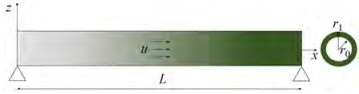Figure  1  Schematic of an axially functionally graded material pipe
 $$E(x)=E_0 f(x)$$ (1a)
 $$E^*(x)=E_0^* f(x)$$ (1b)
 $$\rho(x)=\rho_0 g(x)$$ (1c)
 $$f(x)=1+\left(\alpha_{\mathrm{E}}-1\right)\left(\frac{x}{L}\right)^k$$ (2a)
 $$h(x)=1+\left(\alpha_{\mathrm{E}^*}-1\right)\left(\frac{x}{L}\right)^k$$ (2b)
 $$g(x)=1+\left(\alpha_\rho-1\right)\left(\frac{x}{L}\right)^k$$ (2c)

where αE = ELEO is the elastic modulus ratio, αE* = ELEO* the coefficient of the viscoelastic damping ratio, and αρ = ρLρO the density ratio.E1, E1, ρ1 and EO, EO* and ρO represent the elastic modulus, coefficient of viscoelastic damping and density at the left and right ends of the pipe, respectively. Figure 2 shows the variation of the material properties along the axial under different exponents k when αE=2.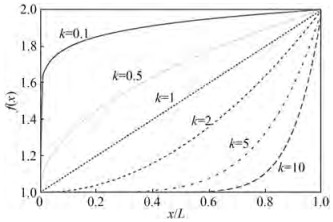Figure  2  Variation of the material properties along the axial under different exponents k (αE=2)

The stress-strain relationship of the Kelvin-Voigt type of viscoelastic material is given by:

 $$\sigma=\left(E(x)+E^*(x) \frac{\partial}{\partial t}\right) \varepsilon$$ (3)

Bending moment is expressed as:

 $$M=\left(E(x)+E^*(x) \frac{\partial}{\partial t}\right) I \frac{\partial^2 w}{\partial x^2}$$ (4)

where w (x, t) is the transversal deflection of the pipe.

Shear force is given by:

 $$S=-\frac{\partial M}{\partial x}=-\frac{\partial}{\partial x}\left[\left(E(x)+E^*(x) \frac{\partial}{\partial t}\right) I \frac{\partial^2 w}{\partial x^2}\right]$$ (5)

The lateral vibration equation of an AFG pipe is written as (Paidoussis and Issid, 1974; Paidoussis, 1987; Paidoussis and Li, 1993),

 $$\begin{array}{r} -\frac{\partial^2}{\partial x^2}\left[\left(E(x)+E^*(x) \frac{\partial}{\partial t}\right) I \frac{\partial^2 w}{\partial x^2}\right]+\frac{\partial}{\partial x}\left[\left(T-A_f p_1\right) \frac{\partial w}{\partial x}\right]= \\ m_f\left(\frac{\partial^2 w}{\partial t^2}+2 u \frac{\partial^2 w}{\partial x \partial t}+u^2 \frac{\partial^2 w}{\partial x^2}+\frac{\mathrm{d} u}{\mathrm{~d} t} \frac{\partial w}{\partial x}\right)+m_f(x) \frac{\partial^2 w}{\partial t^2} \end{array}$$ (6)

where, T is the axial force, Af is the cross-sectional flow area and p1 is the fluid pressure.

Considering that the extension of the pipe due to bending deformation will produce additional axial force:

 \begin{aligned} T-A_f p_1= & T_0-A_f p_o(1-2 v \delta)+m_f \frac{\mathrm{d} u}{\mathrm{~d} t}(x-L) \\ & +\left[E(x)+E^*(x) \frac{\partial}{\partial t}\right] \frac{A_p}{2 L} \int_0^L\left(\frac{\partial w}{\partial x}\right)^2 \mathrm{~d} x \end{aligned} (7)

where, T0 is static tension, and p0 is the pressure of internal flow at x = L.

Substituting Eq-7 into the lateral vibration equation (Eq-6) of the AFG pipe, we have:

 \begin{aligned} & \frac{\partial^2}{\partial x^2}\left[E(x) I \frac{\partial^2 w}{\partial x^2}+E^*(x) I \frac{\partial^3 w}{\partial x^2 \partial t}\right] \\ &+\left[m_{\mathrm{f}} u^2-T_0+A_f p_0(1-2 v \delta)+m_{\mathrm{f}} \frac{\mathrm{d} u}{\mathrm{~d} t}(L-x)\right] \frac{\partial^2 w}{\partial x^2} \\ &-\frac{\partial}{\partial x}\left[\left(E(x)+E^*(x) \frac{\partial}{\partial t}\right) \frac{A_p}{2 L} \int_0^L\left(\frac{\partial w}{\partial x}\right)^2 \mathrm{~d} x \frac{\partial w}{\partial x}\right] \\ &+2 m_{\mathrm{f}} u \frac{\partial^2 w}{\partial x \partial t}+\left[m_{\mathrm{f}}+\rho(x) A_{\mathrm{p}}\right] \frac{\partial^2 w}{\partial t^2}=0 \end{aligned} (8)

Neglecting the pressure p0 and static tension T0, we have:

 \begin{aligned} & \frac{\partial^2}{\partial x^2}\left[E(x) I \frac{\partial^2 w}{\partial x^2}+E^*(x) I \frac{\partial^3 w}{\partial x^2 \partial t}\right] \\ &+\left[m_{\mathrm{f}} u^2+m_{\mathrm{f}} \frac{\mathrm{d} u}{\mathrm{~d} t}(L-x)\right] \frac{\partial^2 w}{\partial x^2} \\ &-\frac{\partial}{\partial x}\left[\left(E(x)+E^*(x) \frac{\partial}{\partial t}\right) \frac{A_p}{2 L} \int_0^L\left(\frac{\partial w}{\partial x}\right)^2 \mathrm{~d} x \frac{\partial w}{\partial x}\right] \\ &+2 m_{\mathrm{f}} u \frac{\partial^2 w}{\partial x \partial t}+\left[m_{\mathrm{f}}+\rho(x) A_{\mathrm{p}}\right] \frac{\partial^2 w}{\partial t^2}=0 \end{aligned} (9)

The following dimensionless parameters are introduced:

 $$\begin{gathered} \xi=\frac{x}{L}, w^*=\frac{w}{L}, \tau=\left(\frac{E_o I}{m_f+\rho_o A_p}\right)^{1 / 2} \frac{t}{L^2}, \\ \alpha_0=\frac{E_0^*}{L^2} \sqrt{\frac{I}{E_0\left(m_f+\rho_0 A_p\right)}}, \\ Q=\frac{A_p L^2}{2 I}, \beta=\frac{m_{\mathrm{f}}}{m_{\mathrm{f}}+\rho_o A_p}, \\ \alpha(\xi)=\frac{\rho(x) A_p}{m_{\mathrm{f}}+\rho_o A_p}, U=u L\left(\frac{m_{\mathrm{f}}}{E_o I}\right)^{1 / 2} \end{gathered}$$ (10)

The dimensionless governing equation is then obtained as (dropping the asterisks of w* for simplicity):

 \begin{aligned} f(\xi) & w^{\prime \prime \prime \prime}+2 f^{\prime}(\xi) w^{\prime \prime \prime}+\left[f^{\prime \prime}(\xi)+U^2+\sqrt{\beta} \dot{U}(1-\xi)\right] w^{\prime \prime} \\ & +\alpha_o h^{\prime \prime}(\xi) \dot{w}^{\prime \prime}+2 \alpha_o h^{\prime}(\xi) \dot{w}^{\prime \prime \prime}+\alpha_o h(\xi) \dot{w}^{\prime \prime \prime \prime} \\ & -Q\left[f(\xi) w^{\prime \prime}+f^{\prime}(\xi) w^{\prime}\right] \int_0^1\left(w^{\prime}\right)^2 \mathrm{~d} \xi \\ & +2 \sqrt{\beta} U \dot{w}^{\prime}-2 \alpha_o Q\left[h(\xi) w^{\prime \prime}+h^{\prime}(\xi) w^{\prime}\right] \int_0^1 w^{\prime} \dot{w}^{\prime} \mathrm{d} \xi \\ & +[\alpha(\xi)+\beta] \ddot{w}=0 \end{aligned} (11)

Here, the prime "'" denotes the spatial derivative in ξ and the dot "∙" denotes the time derivative inτ.

The internal fluid velocity of the AFG pipe is assumed to be sinusoidal and given in the following dimensionless form:

 $$U=U_0(1+\psi \sin (\omega \tau))$$ (12)

whereU0 is the average flow velocity, ψ is the pulsation amplitude, and ω is the dimensionless pulsation frequency.

The boundary conditions for simply-supported ends are:

 $$w(0, \tau)=w(1, \tau)=w^{\prime \prime}(0, \tau)=w^{\prime \prime}(1, \tau)=0$$ (13)

Following the formalism of the generalized integral transform technique, the governing equation of the following auxiliary eigenvalue problem is employed (Cotta et al., 2019; Fu et al., 2019; Li et al., 2020) :

 $$\frac{\mathrm{d}^4 X_i(\xi)}{\mathrm{d} \xi^4}=\mu_i^4 X_i(\xi), 0<\xi<1$$ (14)

with simply supported (SS) boundary conditions:

 $$X_i(0)=X_i^{\prime \prime}(0)=X_i(1)=X_i^{\prime \prime}(1)=0$$ (15)

where the eigenfunctions Xi(ξ) and eigenvalues μi are given by:

 $$X_i(\xi)=\sqrt{2} \sin \left(\mu_i \xi\right), i=1, 2, 3 \cdots$$ (16)
 $$\mu_i=i \pi, i=1, 2, 3 \cdots$$ (17)

The eigenfunctions satisfy the following orthonormal property:

 $$\int_0^1 X_i(\xi) X_j(\xi) \mathrm{d} \xi=\delta_{i j}=\left\{\begin{array}{l} 1, i=j \\ 0, i \neq j \end{array}\right.$$ (18)

The integral transform and inverse pairs are defined by:

 $$\bar{w}_i(\tau)=\int_0^1 w(\xi, \tau) X_i(\xi) \mathrm{d} \xi$$ (19a)
 $$w_i(\xi, \tau)=\sum\limits_{i=1}^{\infty} X_i(\xi) \bar{w}_i(\tau)$$ (19b)

Multiplying the governing equation (Eq-11) by the eigenfunction Xi(ξ) and integrating in ξ ∈ [ 0, 1], the gonvern equation can be rewritten:

 \begin{aligned} & \sum\limits_{j=1}^{\infty}\left[L_{i j} \frac{\mathrm{d}^2 \bar{w}_j(\tau)}{\mathrm{d} t^2}\right]+\sum\limits_{j=1}^{\infty}\left[\alpha_o\left(A_{i j}^* \mu_j^4+C_{i j}\right)+2 U \sqrt{\beta} H_{i j}\right] \frac{\mathrm{d} \bar{w}_j(\tau)}{\mathrm{d} t} \\ & -2 \alpha_o Q \sum\limits_{j=1}^{\infty}\left[N_{i j} \bar{w}_j(\tau)\right] \sum\limits_{j=1}^{\infty} \sum\limits_{k=1}^{\infty}\left[G_{j k} \bar{w}_j(\tau) \frac{\mathrm{d} \bar{w}_k(\tau)}{\mathrm{d} t}\right] \\ & -Q \sum\limits_{j=1}^{\infty}\left[F_{i j} \bar{w}_j(\tau)\right] \sum\limits_{j=1}^{\infty} \sum\limits_{k=1}^{\infty}\left[G_{j k} \bar{w}_j(\tau) \bar{w}_k(\tau)\right] \\ & +\sum\limits_{j=1}^{\infty}\left[\left(A_{i j} \mu_j^4+B_{i j}+U^2 D_{i j}+\sqrt{\beta} \dot{U} E_{i j}\right) \bar{w}_j(\tau)\right]=0 \end{aligned} (20)

where these coefficients defined by:

 $$A_{i j}=\int_0^1 f(\xi) X_i X_j \mathrm{~d} \xi$$ (21a)
 $$A_{i j}^*=\int_0^1 h(\xi) X_i X_j \mathrm{~d} \xi$$ (21b)
 $$B_{i j}=2 \int_0^1 f^{\prime}(\xi) X_i X_j^{\prime \prime \prime} \mathrm{d} \xi+\int_0^1 f^{\prime \prime}(\xi) X_i X_j^{\prime \prime} \mathrm{d} \xi$$ (21c)
 $$C_{i j}=2 \int_0^1 h^{\prime}(\xi) X_i X_j^{\prime \prime \prime} \mathrm{d} \xi+\int_0^1 h^{\prime \prime}(\xi) X_i X_j^{\prime \prime} \mathrm{d} \xi$$ (21d)
 $$D_{i j}=\int_0^1 X_i X_j^{\prime \prime} \mathrm{d} \xi$$ (21e)
 $$E_{i j}=\int_0^1(1-\xi) X_i X_j^{\prime \prime} \mathrm{d} \xi$$ (21f)
 $$G_{i j}=\int_0^1 X_i^{\prime} X_j^{\prime} \mathrm{d} \xi$$ (21g)
 $$F_{i j}=\int_0^1 f(\xi) X_i X_j^{\prime \prime} \mathrm{d} \xi+\int_0^1 f^{\prime}(\xi) X_i X_j^{\prime} \mathrm{d} \xi$$ (21h)
 $$H_{i j}=\int_0^1 h^{\prime}(\xi) X_i X_j^{\prime} \mathrm{d} \xi$$ (21i)
 $$N_{i j}=\int_0^1 h(\xi) X_i X_j^{\prime \prime} \mathrm{d} \xi+\int_0^1 h^{\prime}(\xi) X_i X_j^{\prime} \mathrm{d} \xi$$ (21j)
 $$L_{i j}=\int_0^1[\beta+g(\xi)(1-\beta)] X_i X_j \mathrm{~d} \xi$$ (21k)

As no previous study on nonlinear vibration results of the AFG pipe is encountered in the literature, the hybrid analytical-numerical solution is verified by analyzing the nonlinear vibration of the simply supported homogeneous pipe. Figure 3 shows the bifurcation diagram by the present study compared with that by Ni et al. (2014), for mass ratio β = 0.6, average flow velocityU0 = 4.5, viscoelastic damping coefficientα0 = 0.005, pulsation amplitude ψ = 0.4, and slenderness ratio parameter Q= 5 000. It can be seen that both the displacement amplitude and the bifurcation point are in good agreement with the results presented by Ni et al. (2014). It can be concluded that the proposed GITT solution for the nonlinear dynamics analysis of pipe was successfully verified.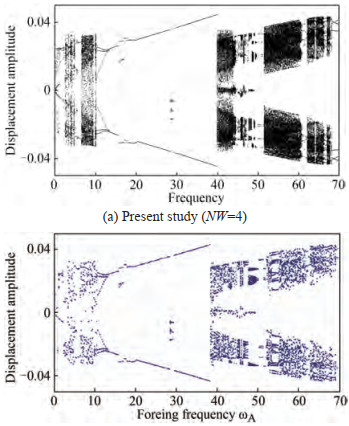Figure  3  Bifurcation diagram of simply supported homogeneous pipe withβ = 0.6, U0= 4.5, α0= 0.005, ψ = 0.4, andQ = 5 000

Convergence analysis has been carried out to analyze the dynamic behavior of the simply supported AFG pipe under pulsating flow. Bifurcation diagrams of the transient displacement at the center of the pipe versus the dimensionless pulsation frequency are shown in Figure 4, with α E = αE∗ = αρ = 2, β= 0.6, U0 = 4.5, α0 = 0.005, ψ = 0.4, and Q = 5 000, calculated with NW= 2, 4, 6, and 8. As can be seen, when the dimensionless pulsation frequency of internal fluid is less than 40, there is no difference between the results with truncation orders NW=2, 4, 6 and 8. However, when the dimensionless pulsation frequency is greater than 40, there is the obvious difference between the bifurcation diagram with NW=2 and higher truncation orders (NW=4, 6 and 8). For truncation orders NW=4, 6, and 8, the variation of the bifurcation diagrams can be negleced. It can be concluded that the present hybrid numerical-analytical model (GITT mdoel) with NW=8 can predict the dynamic behavior of AFG pipes within the selected dimensionless frequency rangeω∈ [ 0, 90 ]. It can be seen from Figure 4 (d) that an AFG pipe (k = 1, αE = αE∗ = αρ = 2) has a complex dynamic behavior in the range of pulsation frequency from 0 to 90, presenting periodic, quasi-periodic, and even chaotic motions.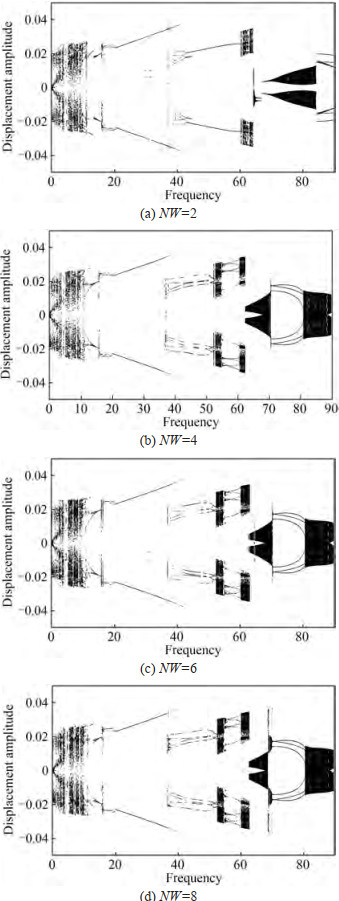Figure  4  Convergence study of bifurcation diagram of AFG pipe with different truncated number (NW) whenβ = 0.6, U0= 4.5, αE= αE∗ = αρ = 2, α0 = 0.005, ψ = 0.4, and Q = 5 000

Figure 5 shows the phase portraits, Poincaré map, power spectra diagram, and time domain diagram for different dimensionless pulsation frequencies shown in Figure 4(d), which can reflect the characteristics of chaotic, quasi-periodic, and periodic motion. Within frequency range ω ∈ [ 0, 10 ], the pipe shows aperiodic (chaotic) motion in a large frequency range, and the power spectra of chaotic motion show a broadband phenomenon without obvious main peaks, as shown by Figures 5 (a) and(b). There is also a small region of periodic motion, in the range of ω ∈ [ 5, 6 ]. In the range of ω ∈ [10, 15 ], the chaotic motion becomes period-1 motion through the period-doubling bifurcation. From ω = 15 to 36.7, the pipe vibration is mainly manifested as period-1 motion. The power spectrum of period-1 motion is a smooth curve with obvious main peaks, shown by Figure 5 (c), but there is a period-doubling motion near ω= 16. For ω ∈ [ 36.7, 52.6 ], the periodic motion is observed. For ω ∈ [ 52.6, 54.7 ] and[ 60.8, 63.6 ], the AFG pipe is mainly manifested as chaotic motion, but between the two ranges, periodic motion is observed. In the range of ω ∈ [ 63.6, 90 ], except for period-3 motion in ω ∈ [ 70.2, 80.7 ], all the other frequencies induce quasic-periodic motion.The power spectrogram of quasic-periodic motion has a wide frequency phenomenon at the same time and an obvious main peak is shown in Figure 5e.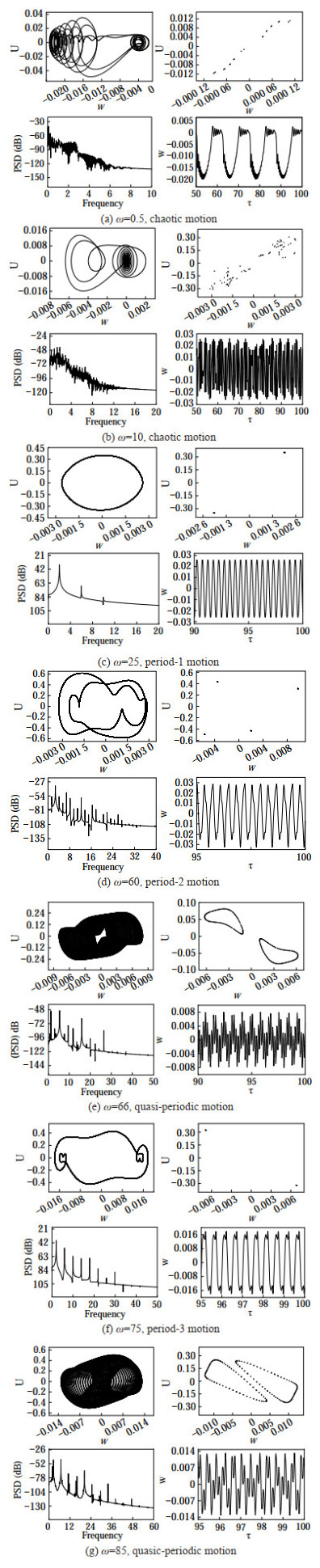Figure  5  Phase portraits diagram, Poincaré diagram, power spectra, and time-domain phase diagram at the midpoint under different pulsation frequencie

Figures 6‒8 show the parametric analysis of the elastic modulus distribution on the dynamic response of the AFG pipe, with the homogeneous density and coefficient of viscoelastic damping (αE∗ = αρ = 1). The elastic modulus ratio α E is taked as 2, and k is takend as 0.1, 0.5, 1, 2, 5 and 10. Figure 6 (a) shows the bifurcation diagram of AFG pipe withk=0.1. In the range of ω ∈ [ 0, 11.7 ], the pipe is mainly manifested as chaotic motion, and there are also areas of partial periodic motion, for instance ω ∈ [ 7.5, 8 ]. In the range of ω ∈[11.7, 15 ], chaotic motion is changed into period-1 motion. In the range of ω ∈[15, 28 ], periodic motion is mainly observed. From ω = 28 to 41.5, it is manifested as period-1 motion. In the range of ω ∈ [ 42.6, 70 ], it is mainly manifested as multi-period motion. From the range of ω= 70 to 90, the static equilibrium state is transformed into periodic motion through Hopf bifurcation. Figure 6(b) shows the bifurcation diagram for k= 0.5. For dimensionless frequencyω=0 to 11.7, it is mainly manifested as chaotic motion, and there is also periodic motion, such as in the range of ω ∈ [ 5, 5.5 ]. Within the range of ω ∈ [11.7, 63.5 ], it is mainly manifested as periodic ω = 10, 50, motion. For dimensionless pulsation frequency ω =63.6 to 72, chaotic motion is transformed into periodic motion. For dimensionless frequency ω=72 to 76.8, it is mainly manifested as quasi-periodic motion. In the frequency range ofω∈ [ 76.85, 90 ], chaotic motion is transformed into periodic motion. For k= 1, as shown in Figure 6 (c), in the range of ω ∈ [ 45.5, 82 ], there exists a large range of chaotic motion, as well as a small range of periodic motion (ω∈ [ 75, 76.3]) and quasi-periodic motion (ω∈ [ 68.8, 69.8 ]). Within the range of ω ∈ [ 82, 90 ], the motion is quasi-periodic. As seen in Figure 6 (c)‒(f), when k ≥ 1the motion state in the low-frequency region (ω ∈ [ 0, 45.5 ]) is stable and does not change with changing k, while there is a large difference in the high-frequency region. With increasing is squeezed, while the periodic and quasi-periodic regions will be extended.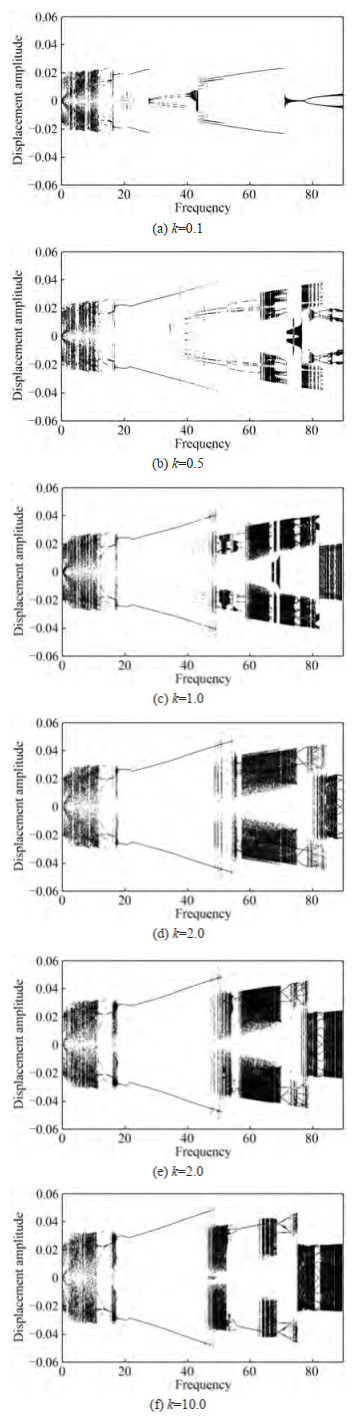Figure  6  Influence of the distribution of elastic modulus on the dynamic behavior of AFG pipe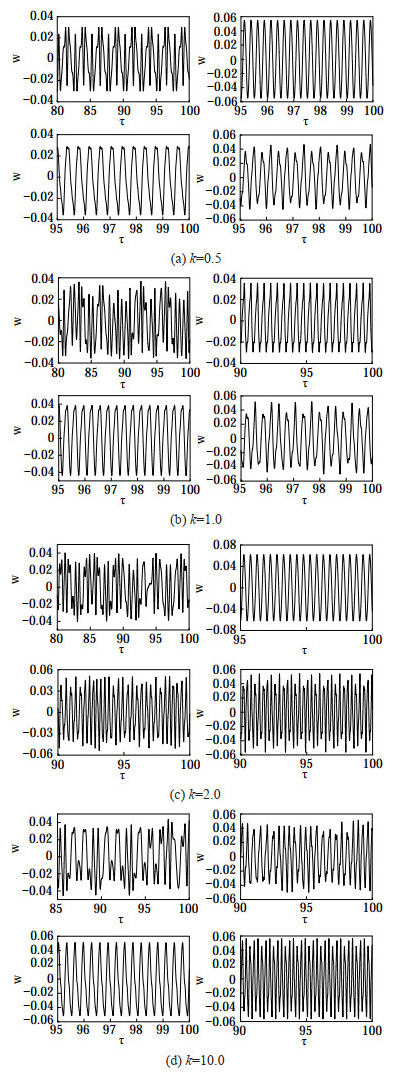Figure  7  Time domain diagram of the system at different k and pulsation frequencies when ω = 10, 50, 60, and 70, repectively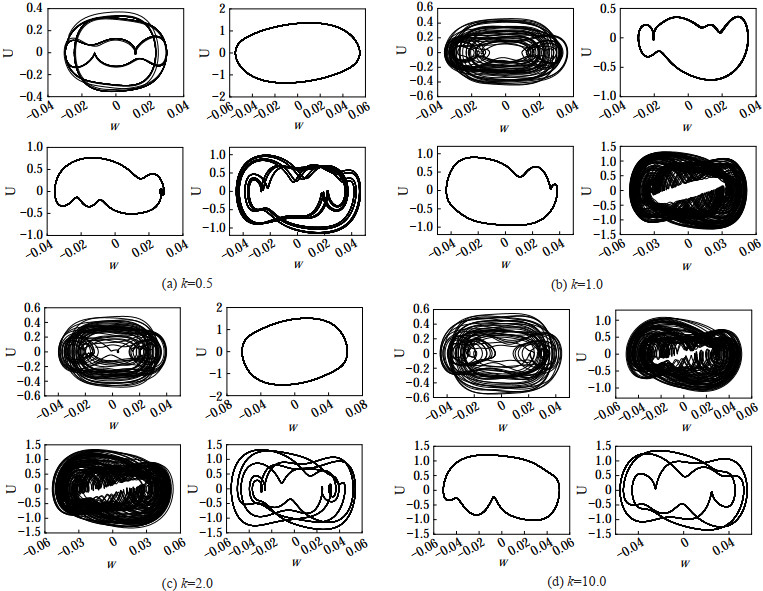Figure  8  Phase portraits of the system at different k and pulsation frequencies when ω = 10, 50, 60, and 70, repectively

It is assumed that the density and elastic modulus of the functionally gradient material pipe are uniform in all directions (αE = αρ = 1), the coefficient of viscoelastic damping ratio αE∗ = 2, and k is taken as 0.1, 0.5, 1, 2, 5, and 10 to analyze the influence of the coefficient of viscoelastic distribution on the dynamic response of AFG pipes.

As shown in Figures 9 (a) and (b), when k < 1, the bifurcation diagram of dimensionless pulsation frequency changes little in the range ofω∈ [ 0, 90 ], and there are great differences mainly in the range of frequency ω ∈ [ 67, 76 ]. When k = 0.1, it shows period-1 motion, and there is also a small range of period-3 motion, as shown in Figure 9 (a). When k = 0.5, period-3 motion is transformed into period-1 motion, as shown in Figure 9 (b). When k= 1, the motion is transformed from multi-periodic motion to period-1 motion, as shown in Figure 9 (c). When k > 1, the region with great variation in the bifurcation diagram is within the range of frequency ω ∈ [ 50, 60 ] andω∈ [ 70, 90 ]. Withinω∈ [ 50, 60 ], when k = 2, it mainly manifests as multi-periodic motion. When k= 5 and k = 10, chaotic motion is mainly manifested in a larger range. When the dimensionless frequency increases from ω=70 to 90, the range of quasi-periodic motion will increase gradually with increasing k. It can be seen that the k has a great influence on the motion state at high frequency, and its influence on the motion state at low frequency can be neglected.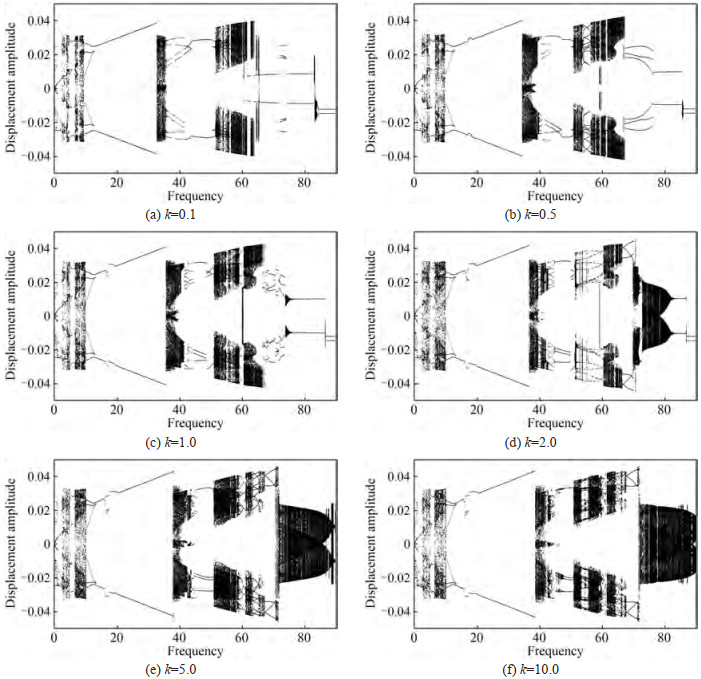Figure  9  Influence of the coefficient of viscoelastic damping distribution on the dynamic behavior of AFG pipe

It is assumed that the elastic modulus and coefficient of viscoelastic damping of AFG pipe are nonuniform (αE= αE∗ = 1), the density ratio αρ = 2, and k is taken as 0.1, 0.5, 1, 2, 5, and 10 to analyze the influence of density distribution on the dynamic response of the pipe. Figure 10 (a) shows the bifurcation diagram for AFG pipe with k= 0.1. In the range ofω∈ [ 0, 10 ], the pipe is in chaotic motion, but there are also a few pulsation frequencies such as ω ∈ [ 4.5, 6 ] that are in periodic motion. Within frequency range ω ∈ [ 9.6, 11.6 ], it appears as period-3 motion. When the frequencyωincreases from 11.6 to 34.4, the period-1 motion appears, but a sudden change occurs at the dimensionless pulsation frequency of 12.6, changing from period-1 motion to period-2 motion and lasting to the frequency of 13.6. In the range of ω ∈ [ 34.4, 61.5 ], it is mainly manifested as chaotic motion, but periodic motion and quasi-periodic motion can also be observed. Within frequency range ω ∈ [ 61.5, 90 ], it is mainly manifested as quasi-periodic motion, and there is also a small range of periodic motion.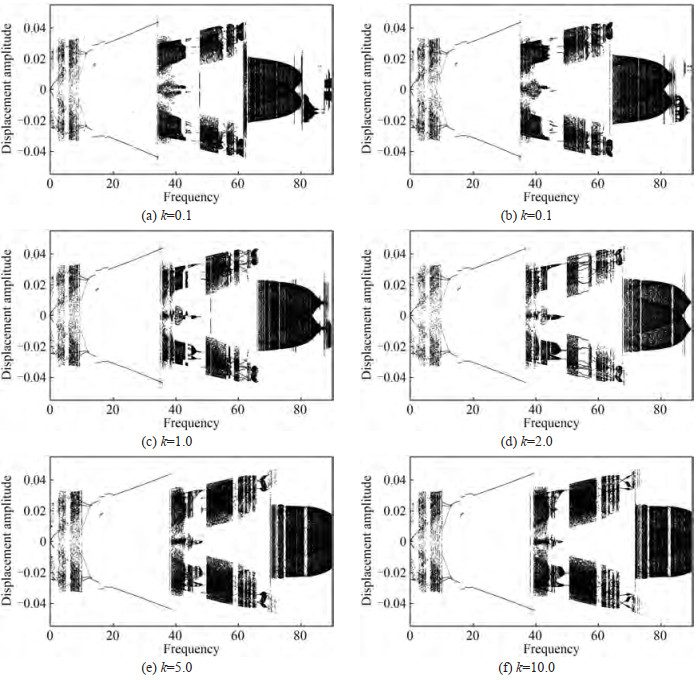Figure  10  Influence of density distribution on the dynamic behavior of AFG pipe

It can be seen from Figure 10 that when the dimensionless pulsation frequencyω is less than 34.4, the effects of the material variation index k on the dynamic response of AFG pipes can be ignored. However, when ω > 34.4, with increasingk, the frequency range of period-1 motion will gradually extended, and the chaotic and quasi-periodic motion frequency regions will not be changed, but the frequency of chaotic and quasi-periodic motion will gradually increase. Therefore, it can be concluded that the k has almost no effect on the motion state under low frequency range (ω ∈ [ 0, 34.4 ]). However, in the high frequency region, with increasing k, the motion state will not change, but the starting frequency at which each motion state occurs is increased.

The nonlinear dynamics of AFG pipe conveying pulsating flow is studied by using the generalized integral transform technique. The effects of the elastic modulus, density and coefficient of viscoelastic damping distribution on the dynamic behavior of the pipe are investigated, with the following main conclusions.

The distribution of the elastic modulus has an important influence on the motion state of the system. When the index of material variation k < 1, it has a greater influence on the motion in both low-frequency and high-frequency regions. However, when k > 1, it has a greater effect on the motion only at high frequencies, with negligible effects in the low frequency region.

The distribution of density has little effect on the motion state of the system, with k having a great influence on the motion state at high frequency, but almost no influence on the motion state at low frequency.

The distribution of the viscoelastic damping coefficient has an important effect on the motion state of the system. In the low frequency region, the k has almost no effect on the motion state. With the increase in k, the motion state at high frequency region will not change, but the starting frequency at which each motion state occurs is increased.

The present method can be extended to the analysis of on nonlinear dynamics of viscoelastic AFG pipe conveying pulsating fluid with other classical boundary conditions, such as clamped and free ends, or more general elastic boundary conditions such as rotationally restrained ends. It can also be extended to the analysis of linear and nonlinear stability of mooring cables or catenary risers using large-deflection Euler-Bernoulli beam models.

Competing interest  The authors have no competing interests todeclare that are relevant to the content of this article.
• Figure  1   Schematic of an axially functionally graded material pipe

Figure  2   Variation of the material properties along the axial under different exponents k (αE=2)

Figure  3   Bifurcation diagram of simply supported homogeneous pipe withβ = 0.6, U0= 4.5, α0= 0.005, ψ = 0.4, andQ = 5 000

Figure  4   Convergence study of bifurcation diagram of AFG pipe with different truncated number (NW) whenβ = 0.6, U0= 4.5, αE= αE∗ = αρ = 2, α0 = 0.005, ψ = 0.4, and Q = 5 000

Figure  5   Phase portraits diagram, Poincaré diagram, power spectra, and time-domain phase diagram at the midpoint under different pulsation frequencie

Figure  6   Influence of the distribution of elastic modulus on the dynamic behavior of AFG pipe

Figure  7   Time domain diagram of the system at different k and pulsation frequencies when ω = 10, 50, 60, and 70, repectively

Figure  8   Phase portraits of the system at different k and pulsation frequencies when ω = 10, 50, 60, and 70, repectively

Figure  9   Influence of the coefficient of viscoelastic damping distribution on the dynamic behavior of AFG pipe

Figure  10   Influence of density distribution on the dynamic behavior of AFG pipeclick to enlarge
Figures(10)
##### Publishing history
• Rev Recd Date:  13 July 2022

### 目录/

• Share
•Scan QR code with wechat

Share with friends and circles of friends